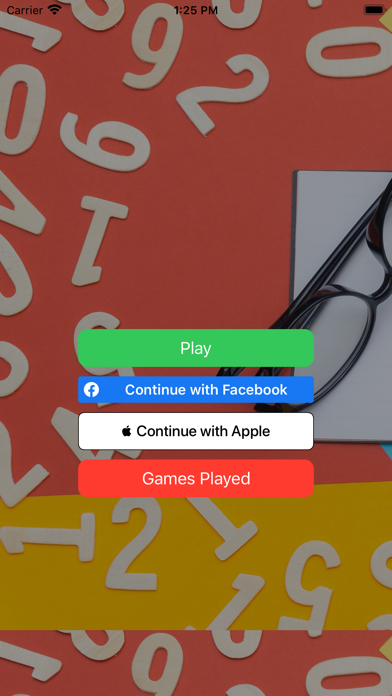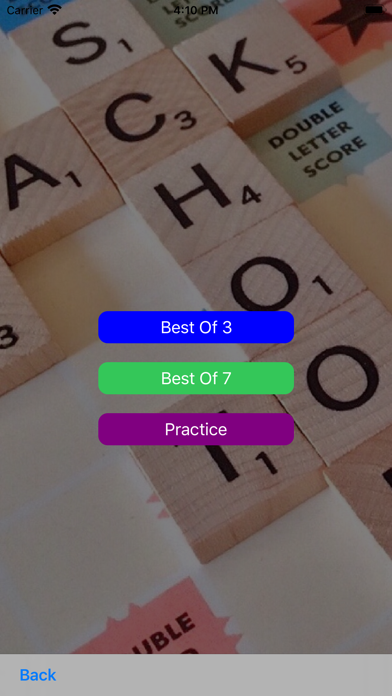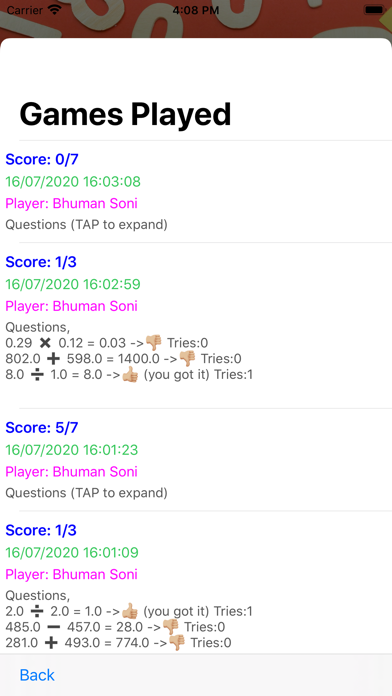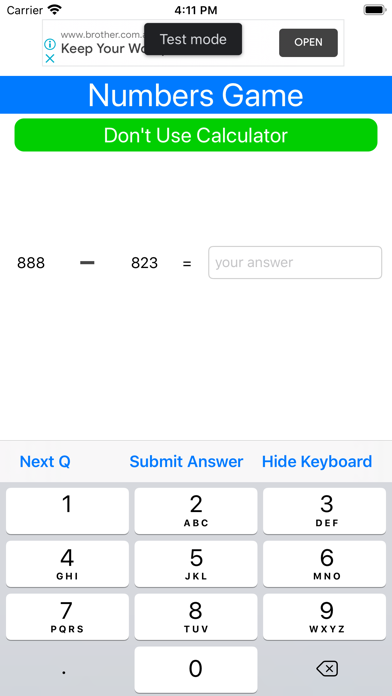# Numbers Game: Calculate Faster

## iOS iPhoneGames

Free was 0.99

This is a simple game with the sole purpose of solving simple math questions without using the calculator. In our day to day life, we see several
math problems present itself e.g. "I will earn a \$987 at the end of the week, after I pay \$323 in taxes, will I have enough to buy my flight tickets this week?" or so on and so forth. We see simple math problems present themselves as we go about our day to day life and we can save ourselves time if we don't need to manually type it in the calculator every time.

One of the trickiest things to solve are multiplying decimal values i.e. 0.32 x 0.43 = ? The answer is 0.1376 but it can take a while to get to. I mean it's all about multiplying 32 x 43 which is 1376 and then moving the answer 4 decimal places which makes it 0.1376.

Even simple subtraction problems e.g. take 997 - 613 = 384. Simple, one of the quicker ways to do this would be to subtract, 997 - 600 which would give us 397, then subtract the remaining 13 i.e. 397 -13 to get 384.

One of the features of this app is that it keeps track of the problems you solved and how long did you take to solve them? You can see the previous outcomes of your games played in the results view. There you can see, what the problem was, the time (seconds) you took to solve it and the date and time when you attempted to solve that problem . This way you can track your progress and identify areas that need improvement.

So use this app to be able to add, subtract, multiply etc, faster than a lot of people you know.

Call it, Numbers Game, Game of Numbers, Simple Numbers, Numerical Aptitude Helper, or anything else, play this game to improve your calculation efficiency.

••••